## Chebyshev Differential Equation(1)

for. The Chebyshev differential equation has regular Singularities at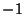, 1, and. It can be solved by series solution using the expansions(2)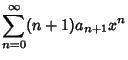(3)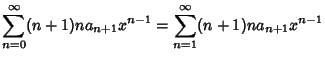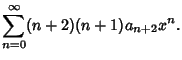(4)

Now, plug (2-4) into the original equation (1) to obtain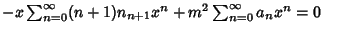(5)(6)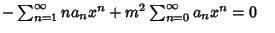(7)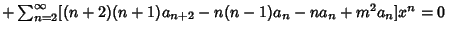(8)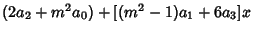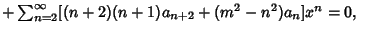(9)
so(10)(11)(12)

The first two are special cases of the third, so the general recurrence relation is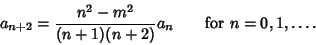(13)

From this, we obtain for the Even Coefficients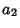(14)(15)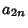(16)

and for the Odd Coefficients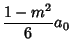(17)(18)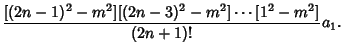(19)

So the general solution is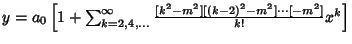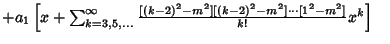(20)

Ifis Even, thenterminates and is a Polynomial solution, whereas ifis Odd, thenterminates and is a Polynomial solution. The Polynomial solutions defined here are known as Chebyshev Polynomials of the First Kind. The definition of the Chebyshev Polynomial of the Second Kind gives a similar, but distinct, recurrence relation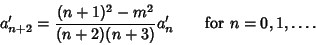(21)